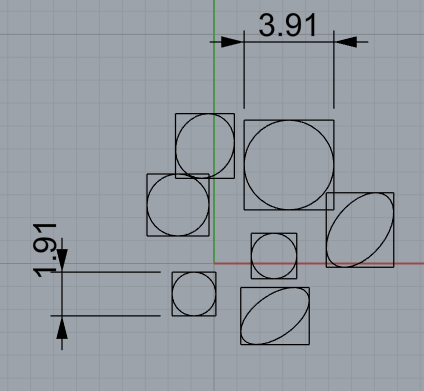# How to get size (diamater) distribution

I would like to create a script that will loop through the domain(see attached) and get the size (diameter/bounding box) for each shape.

Any help would be appreciated.

Eric

Bounding box is not too hard to do, but the question is what do you want to do with the info obtained afterward? --Mitch

Thanks, Mitch
The objective is to generate a size distribution curve where the sizes will be binned into about 100 bins and the frequencies for each obtained.

Size as in bounding box area? For diameter you would need a minimum bounding circle which would take some iteration. I don’t think there are any Rhino functions that will find this for you. Are you constraining the input objects to 2d curves?

Size as in maximum bounding box dimension. Yes, will constrain the input objects to 2D curves.

This works here. Will give you a list of max lengths that you can use to feed your histogram. I think it should work for any 3d rhino doc object but I didn’t test. Also I guess I didn’t ask if you meant python or rhino script. This is python.

``````import rhinoscriptsyntax as rs
import scriptcontext as sc
import Rhino as R

def get_max_bb_dims(guids):
max_lengths = []
for guid in guids:
geo = rs.coercegeometry(guid)
if geo:
bbox = geo.GetBoundingBox(R.Geometry.Plane.WorldXY)
x_length = abs(bbox.Diagonal.X)
y_length = abs(bbox.Diagonal.Y)
z_length = abs(bbox.Diagonal.Z)
max_length = max(x_length, y_length, z_length)
max_lengths.append(max_length)
return max_lengths

# get the curve guids
guids = rs.GetObjects('select 2d curves in XY plane')
# dims will be a list of max bbox dimensions
dims = get_max_bb_dims(guids)
# print out min/max dims for a check
print('minimum dim: {}, maximum dim: {}'.format(min(dims), max(dims)))
``````

Output:
minimum dim: 1.9131, maximum dim: 3.9119

Source curves (and bounding box rectangles):Assumes the curves are in XY plane. No error handling.